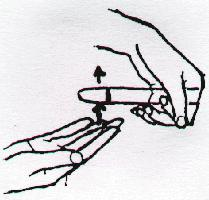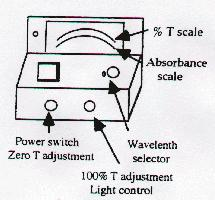Tehilla Rieser                                                                                                        Bronx High School of  Science
Summer Research Program for Science Teachers                                              1998

How can we calculate an equilibrium constant? [9-12 Content Standard B- Chemical reactions]

Objectives:  Students should be able to:

- Explain how the relative equilibrium quantities of reactants and products are shifted by changes in temperature, pressure, or  concentrations of substances in the equilibrium reaction.
- Calculate equilibrium constant from equilibrium concentrations.
- Explain the relationship between absorption of light and concentration.
- Identify and explain the function of each part of spectrophotometer. [9-12 Content Standard A- Understandings about scientific inquiry]

1. The concept of equilibrium
Once a chemical reaction achieves equilibrium, the concentrations of all reactants and products remain constant, and some  amount of each remains.

2.    The equilibrium constant

• The law of mass action expresses the relative concentrations of reactants and products in terms of a quantity called Keq .
• The value of Keq is only affected by temperature.
• Changes in concentrations do not affect Keq.
• Keq must be positive.
• When Keq is much larger than 1, at equilibrium the concentration of product(s) is far greater reactants.  When Keq is much smaller than 1, at equilibrium the concentration of reactants is much greater than products.

3.    Le Chatelier's principle

If a system is in equilibrium and the concentration of reactant(s) and/or product(s) is/are changed then the reaction will no longer be in equilibrium.  However, the concentration of all substances will redistribute and a new equilibrium will be achieve.  Although the concentrations of reactants and products present at the second equilibrium will now be different, the value of Keq, which is calculated from the law of mass action, will remain the same as long as temperature has been kept constant.

[Content Standard Unifying Concepts- Systems, order, and organization]

[Content Standard Unifying Concepts- Equilibrium]

4.    Beer-Lambert law

There is a linear relationship between absorbance and concentration when the law is obeyed. [Content Standard Unifying Concepts- Change, constancy, and measurement] (However, since deviations from this law sometimes occur, calibration curves should be constructed.)  By comparing the absorbance of a solution of   unknown concentration with that of a known standard, we can calculate the unknown concentration of the colored product.

An equilibrium table is then used to calculate the concentrations of all other species in reaction equation.  Using the law   of mass action, Keq can now be determined.

5.    Spectrophotometer: Schematic diagram• Light bulb to produce white light
• Diffraction grating to "break up" white light
• Slit to allow only narrow wavelength bands to enter
Rotation of grating selects portion of spectrum illuminating sample
• Sample chamber holds the cuvette
• Meter has scale calibrated in transmittance (top) and absorbance (bottom)

[9-12 Content Standard E- Understandings about science and technology]

DETERMINATION OF AN EQUILIBRIUM CONSTANT

OBJECTIVES:

(a) To develop an understanding of the relationship between the absorbance of light and concentration.
(b) To determine an equilibrium constant from spectrophotometric data.

MATERIALS:

Glassware: Five 6" test tubes, five 4" test tubes, pipettes, small beakers, four 10 mL graduated cylinders.
Stock solutions: 10 mL of 0.10M Fe(NO3)3, 20 mL of 0.01M Fe(NO3)3, 10 mL of 0.00 1M KSCN.
Equipment: Spec-20 with "blank". [Teaching Standard D- Make accessible science materials]

INTRODUCTION:

Any chemical reaction will eventually proceed to a state of dynamic equilibrium, in which the reactants and products are present in definite concentrations.  In some cases, the equilibrium will be attained only after nearly all of the reagents have been consumed and converted to reaction products.  Such a reaction is said to "go to completion."  Often a substantial fraction of the original reagent concentration remains after equilibrium has been achieved.

This experiment will investigate the reaction of ferric ions with thiocyanate ions and the condition of equilibrium which results.  The concentration of reactants and products remaining at equilibrium will be related to each other by a mathematical expression involving the equilibrium constant of the reaction.  The two reactant ions, which are nearly colorless, react to form a red-colored complex, the ferrithiocyanate ion.

Fe3+  + SCN -                         FeSCN2+
colorless                             red complex

The higher the concentration of the FeSCN2+, the more intense is the red color of the solution.  Thus, the color of the solution allows the experimenter to infer the amount of FeSCN2+ present, either by observing the solution visually, or more accurately, by using an absorption spectrophotometer. [9-12 Content Standard B- Chemical reactions]

The actual concentration of the FeSCN2+ complex present at equilibrium in a solution may be obtained by comparing its color with standard solution, in which the concentration of FeSCN2+ is known.  To prepare the standard solution, the complex is formed from Fe3+ and SCN- ions, using a very high concentration of Fe3+ ions.  Then, according to Le Chatelier's Principle, the enormous excess of Fe3+ concentration will drive the equilibrium so that virtually all of the SCN- ions originally present will have reacted to form the FeSCN2+ complex.

PROCEDURE:

Mixing Solutions in Test Tubes1. Grasp the top of the test tube firmly (but not tightly) by curling your index finger and thumb around the neck of the test tube (these instructions apply to right-handed individuals) with your left hand.
2. With a rapid motion, slap the bottom of the test tube with the outstretched fingers of your right hand.  Your fingers should slide past the bottom of the tube after slapping it.  Repeat this motion periodically (about once every second).  If this is done correctly, a vigorous vortex motion is imparted to the liquid in the tube.

To prepare a standard solution, pipet 2 mL of 0.0010 M K SCN into a medium size (1.8 x 15 cm) test tube.  Then, add by pipet 8 mL of a fairly concentrated (0.10 M) Fe (NO3)3 solution (Caution: The Fe (NO3)3 solutions are very corrosive.)   The addition of the Fe (NO3)3 solution will result in such a high concentration of  Fe3+ ions in solution so as to ensure that virtually all of the SCN- ions have reacted to form the complex.  Consequently, each mole of SCN- ions initially added to the solution will be converted to one mole of   FeSCN2+ complex.  The total volume of the standard solution however, is five times larger than the initial volume of K SCN solution which was added.   Thus, this concentration FeSCN2+ complex in the standard solution will be one-fifth the concentration of the K SCN reagent solution.  After recording the exact concentration of K SCN listed on the label of the stock solution, determine the concentration of the complex present in the standard.  (See note p2).

As various solutions are prepared, differing in the amount of Fe3+ added, it will be possible to determine the concentration of  FeSCN2+ complex formed at equilibrium by comparing the intensity of color of the solution with that of the standard.  This is done most accurately by measuring the amount of light absorbed by the FeSCN2+ complex present in each solution, using an absorption spectrophotometer.  The absorbance of light by each solution will be proportional to the concentration of FeSCN2+ present at equilibrium.  For example, when the absorbance of the solution in test tube one is compared with the absorbance of the standard, then.

[FeSCN2+ ] test tube #1 = Absorbance  test tube # 1
[FeSCN
2+ ]
standard             Absorbance standard

In this manner, several solutions will be prepared, differing only in the amount of  Fe3+ initially added.  The absorbance of each solution can be compared with the absorbance of the standard in order to determine the concentration of  FeSCN2+ present at equilibrium.   Prepare the solutions as described in the table below.  Note that the same amount of SCN- is initially pipetted into each solution, and that water is added as indicated so that all solutions will have the same volume.  Label all test tubes before preparing the solutions.  Use 0.010 M Fe(NO3)3.

 Test Tube #1 Test Tube #2 Test Tube #3 Test Tube #4 0.0010M SCN- 2.0 mL 2.0 mL 2.0 mL 2.0 mL 0.010M Fe3+ 8.0 mL 6.0 ML 4.0 mL 2.0 mL water 0.0 mL 2.0 mL 4.0 mL 6.0 mL

Once the solutions have been prepared, the concentration of FeSCN2+ at equilibrium in each test tube is determined by comparing the absorbance of light of each solution with that of the standard.

 Operating Procedure Plug machine and allow it to warm up for 5 minutes. Set the wavelength of the spectrophotometer to 525 nm.Adjust the 0% transmission control. Insert "blank" (test tube with water) to adjust the 100% control. Measure the absorbance of each solution; record results below.

From absorbencies of the solutions relative to the standard, and from the known concentration of FeSCN2+ in the standard, calculate the concentration of FeSCN2+ complex present at equilibrium in each solution.

 Test Tube Absorbance [FeSCN2+ ]Eq Standard #1 #2 #3 #4

Once the concentration of  FeSCN2+ produced in each test tube has been determined, it is a simple matter to find the concentration of unreacted Fe3+ and SCN- ions remaining in each solution.

____________________________________

Note:  The number of moles of  Fe3+  ions present in the standard solution is 400 times greater than the number of moles of   SCN-  initially present.  Clearly, the Fe3+ is present in large excess, so that the amount of  FeSCN2+ complex which may be formed is limited by the amount of  SCN- initially added.  We might suspect then, that even if the ferric ion concentration was much lower than, but still in excess of, the amount of  SCN- initially added, the reaction would still produce the same concentration of  FeSCN2+ and have the same color.  This will not be the case however, since the concentration of Fe3+   ions will then be insufficient to drive the equilibrium towards completion, and much of the K SCN will remain unreacted in the solution.  In fact, it will become very apparent that, as the concentration of ferric ions is decreased, progressively less of the SCN- ions initially added will be converted into the complex (even though the amount of  Fe3+ is still in excess of that required to react with all the SCN-).  The lower concentration of the complex present at equilibrium will result in a paler color of this solution than in the standard.

CALCULATIONS:

Calculate and record the "initial concentration" of the reactants. Take into account the concentration of the stock solutions used, and the dilution factor for each reagent added occupying the entire 10 mL volume of each solution.

Now, we consider the reaction to have occurred, and that some of the Fe3+ and SCN- ions initially present had reacted to form the complex.  The balanced equation for the reaction shows that for every mole of FeSCN2+ complex which has formed, one mole of  Fe3+ and one mole of SCN- have reacted.  Since the concentration of  FeSCN2+ present at equilibrium has already been determined for each solution, the amount of Fe3+ and SCN- consumed by the reaction is known.  The concentration of  Fe3+ and SCN- which remain at equilibrium is simply the difference between the initial concentration and the concentration which has reacted.  Summarize the concentrations of reactants and products present at equilibrium.

 Standard Fe3+ SCN- FeSCN2+
 Initial: Change: Equilibrium:

 Test Tube 1 Fe3+ SCN- Fe(SCN)2+ Test Tube 2 Fe3+ SCN- Fe(SCN)2+
 Initial: Initial: Change: Change: Equilibrium: Equilibrium:

 Test Tube 3 Fe3+ SCN- Fe(SCN)2+ Test Tube 4 Fe3+ SCN- Fe(SCN)2+
 Initial: Initial: Change: Change: Equilibrium: Equilibrium:

Although the concentration of reactants and products present at equilibrium are different for each solution, the concentrations present in each solution are related by the mathematical relationship,

[FeSCN2+]Eq      =   K                   where K is the equilibrium constant of the reaction of 25ºC
[Fe3+ ]Eq   [SCN- ]Eq

For each test tube, calculate the equilibrium constant and summarize your results below.

 Test Tube Calculated K #1 #2 #3 #4

Average value K = __________________                     Standard Deviation = ________________________

[9-12 Content Standard A- Use mathematics to improve communication]

WRITE UP:  All your calculations must be included in your lab report.